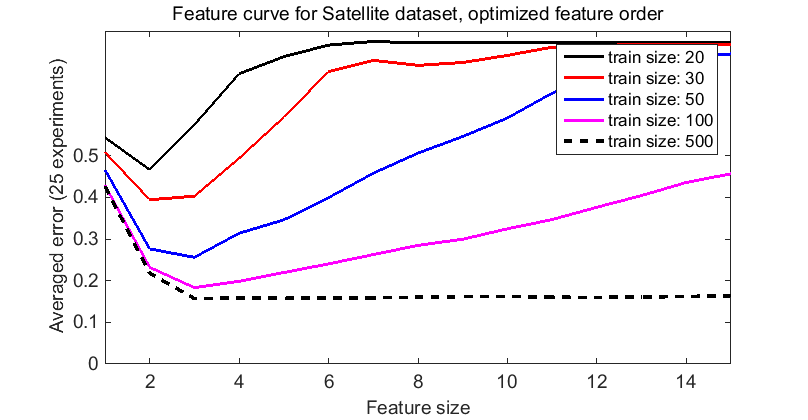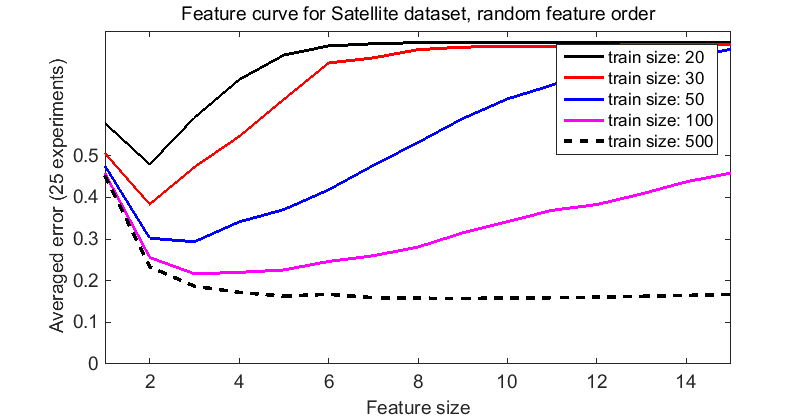# fcurves

Feature curves for Bayes-Normal, on the Satellite dataset. Average over 25 repetitions

PRTools and PRDataSets should be in the path. See http://37steps.com/prtools for more. Download m-file from Download the m-file from here

## Initialization

```delfigs
a = satellite;
a = setprior(a,getprior(a));
w = featself(a,'maha-s');
trainsize = [20 30 50 100 500];
iter = 25;
```
```
*** You are using one of the datasets distibuted by PRTools. Most of them
*** are publicly available on the Internet and converted to the PRTools
*** format. See the Contents of the datasets directory (HELP PRDATASETS)
*** or the load routine of the specific dataset to retrieve its source.

PR_Warning: getprior: fcurves: No priors found in dataset, class frequencies are used instead
```

## Create feature curves for optimized feature ranking

```x = a*w;
randreset;
e1 = cell(1,numel(trainsize));
% prwaitbar calls are just used for reporting progress and may be skipped
[n,s,count] = prwaitbarinit('Processing %i curves:',2*numel(trainsize));
for j=1:numel(trainsize)
e1{j} = clevalf(x,remclass(2)*qdc([],[],1e-6),[1:15],trainsize(j),iter,[],testd);
count = prwaitbarnext(n,s,count);
end
plote(e1,'nolegend')
legend('train size: 20','train size: 30','train size: 50','train size: 100','train size: 500')
title('Feature curve for Satellite dataset, optimized feature order')
fontsize(14)
set(gcf,'position',[ 680 558 808 420]);
```
```PR_Warning: normal_map: Singular case, pseudo-inverse of the covariance matrix is used.
```## Create feature curves for randomized feature ranking

```figure
randreset;
x = a(:,randperm(size(a,2)));
e2 = cell(1,numel(trainsize));
for j=1:numel(trainsize)
randreset;
e2{j} = clevalf(x,remclass(2)*qdc([],[],1e-6),[1:15],trainsize(j),iter,[],testd);
count = prwaitbarnext(n,s,count);
end
plote(e2,'nolegend');
legend('train size: 20','train size: 30','train size: 50','train size: 100','train size: 500')
title('Feature curve for Satellite dataset, random feature order')
fontsize(14)
set(gcf,'position',[ 680 558 808 420]);
```